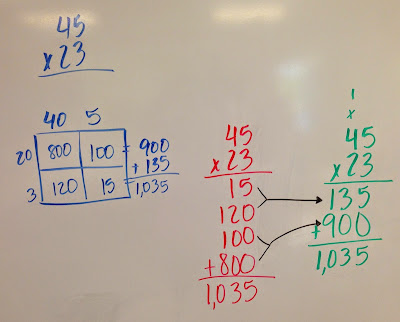## Monday, October 24, 2016

### Moving to the Standard Multiplication AlgorithmThe progression from matrix box to standard algorithm
This week we moved to the standard American algorithm for multiplication. Parents are always thrilled when we get to this, as this is the way they learned! We began by looking at our area model (in blue), and discuss what places were being multiplied during the process. The tens places of both numbers are multiplied together, then the tens of the first number is multiplied by the ones of the second number. Next the ones of the first number is multiplied by the tens of the second number. Finally, the ones of the first number is multiplied by the ones of the second number. Sounds confusing, right?! So we begin to discuss what this would look like vertically (in red). Then we discuss the traditional algorithm (in green), and make connections between all three strategies. This really helps your children understand the place value involved in the algorithm! Woohoo!